# Two New Devices Help Reinvent the Signal Generator. Part 2

David Hunter, Analog Devices

Analog Dialogue

Part 1.

For this design, the differential output amplitude will be ±1.0 V. With factory default settings, the internal 500 Ω resistors, and maximum gain, the input amplitude would have to be 100 µV. Adding resistors to the direct-input pins allows the designer to adjust this requirement. The gain range, as determined by the input resistors, is: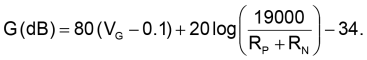(2)

Using 40.2-kΩ resistors at each input provides a good balance between noise power and input attenuation. With VG = 1.1 V (maximum gain), the gain is: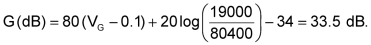(3)

In this case, the differential input only needs to be 21 mV.

When VG = 0.1 V, the gain is: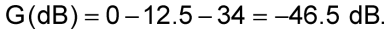(4)

For the same 21 mV input, the output will be about 100 µV.

Factoring in the total gain of the AD8130 and the ADA4870, which amounts to 24.1 dB, the output amplitude of the ADA4870 will range from 1.6 mV to 16 V. After the resistive pad and autotransformer, the voltage on the output will range from 2 mV to 20 V.

Interfacing the AD8338 to a device such as a DDS requires accounting for the antialiasing and input attenuation. For example, the differential output AD9834C DDS  requires 200-Ω resistors to ground for proper swing. Each output produces only half of the sine-wave signal, as shown in Figure 6.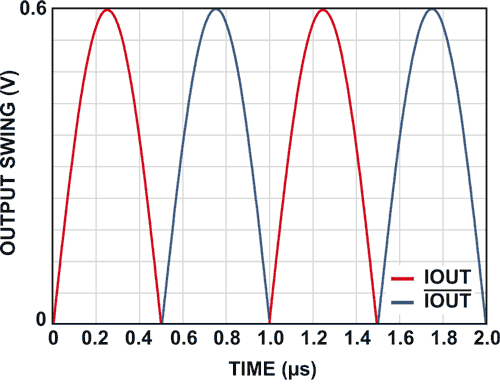Figure 6. Output swing of the AD9834C IOUT and /IOUT. Aliasing artifacts are not shown.

Each output peaks at 0.6 V, making an effective input of ±0.6 V. The required attenuation is 26 dB. With 200-Ω resistors, the attenuation is easily achieved by creating a simple resistive divider. Since the signal does not swing uniformly, the peak of the signal should reach the expected attenuated value.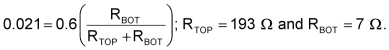(5)

Using standard 6.98-Ω and 191-Ω resistor values will contribute 0.7% error.

Finally, some antialiasing will be necessary. With a 75-MSPS sample rate, the Nyquist-rate output would be 37.5 MHz, which exceeds the 20-MHz bandwidth of this design. Setting the antialiasing pole at 20 MHz, the required capacitor is: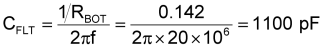(6)

This is a standard value, so the complete input network is as shown in Figure 7: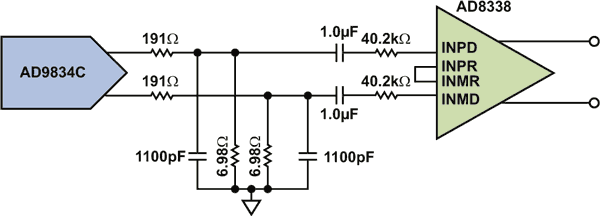Figure 7. DDS + attenuation and filter network + AD8338.

This stage was constructed and measured. The overall variation was within ±0.6 dB, as shown in Figure 8.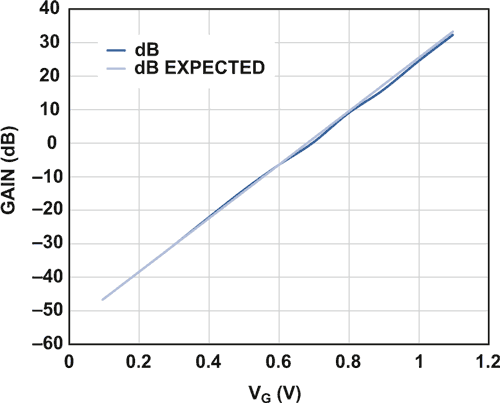Figure 8. Calculated vs. measured gain for AD8338 configuration.

With the single-ended output provided by the AD8130, the ADA4870 will perform the final gain-of-10. Two resistors are needed to set this gain, and the stage is stable without external compensation. The only work that will be left is shaping the output network to conform to the application’s need. There are three general implementations:

1. Direct output from amplifier to 50 Ω
2. Padded, autotransformer output to 50 Ω
3. Unpadded, autotransformer output to 50 Ω

For a direct output, the amplifier output is tied directly to the output connector, without any network to transform the source, as shown in Figure 9. Perfect for the true-dc-connected source, this method doesn’t use the device’s full potential, yet still does better than the typical signal generator’s 10-V output amplitude. In this case, the maximum peak power will be 5.12 W.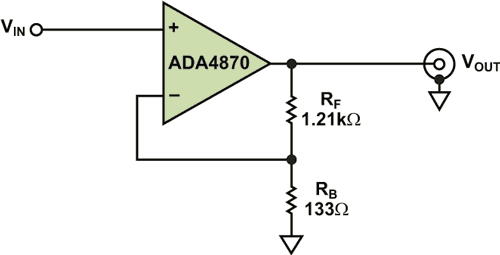Figure 9. Connections for direct output drive.

With the padded design, the 16-Ω load is split between an 8-Ω series pad, and the filtered 1.5:1 autotransformer, as shown in Figure 10. In this mode, the low impedance of the design enables the designer to use 6.25× smaller values for the inductors than those used for 50-Ω designs. The low-pass filter and autotransformer convert the effective 8-Ω source impedance to a well matched 50 Ω. While the total peak output power will be 8 W, this design method is best suited to applications that need a matched 50-Ω source where reflections might be of concern, such as when long transmission lines are involved.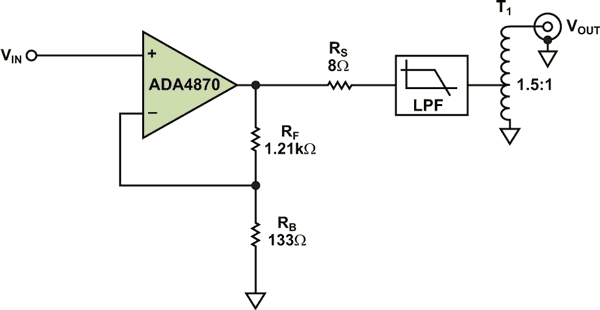Figure 10. ADA4870 connections for padded-output design.

Input impedance appears as 50 Ω to any reflections within the band of interest

The last option, and probably the most useful for signal generators, skips the use of the 8-Ω pad, and permits twice the output power. An LC ladder filter is still recommended, as shown in Figure 11, but the ladder values will be 3.125× smaller than values which would be used for a 50-Ω system (design to 16-Ω nominal impedance). In this case, the autotransformer will use a 0.77:1 ratio. In this mode, the peak sine-wave output amplitude will be 28.3 V, and the ADA4870 will drive approximately 16 W into a 50-Ω load (8-W rms or 39 dBm).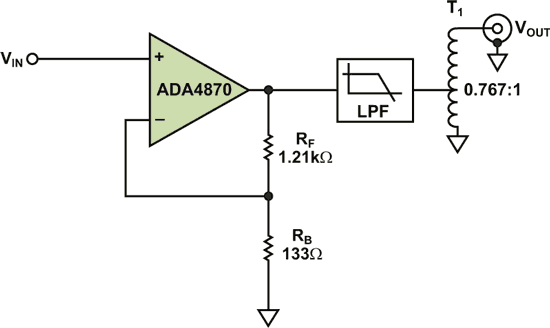Figure 11. Connections for optimal power output for driving 50-Ω loads.

The Total Solution

In the real world, simulations and equations are meaningless if they don’t match the real world. It is thus important to build a complete system and measure its performance against expectations. Figure 12 shows the schematic of an actual padded-output design.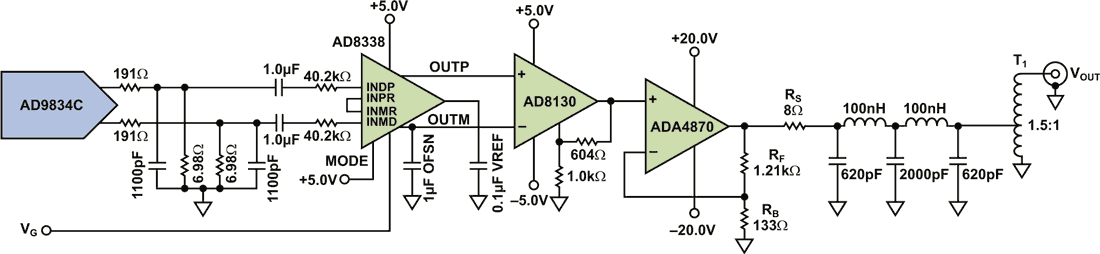Figure 12. Simplified, complete schematic.

Figure 13 shows measured results without a filter. The system has a ±1-dB gain conformance worst case up to the 2.75 W rms (5.5-W peak) output power (P1dB compression point at 34 dBm). Most notably, the total gain range exceeded 62 dB, offering 16 dB more range than many standard generators.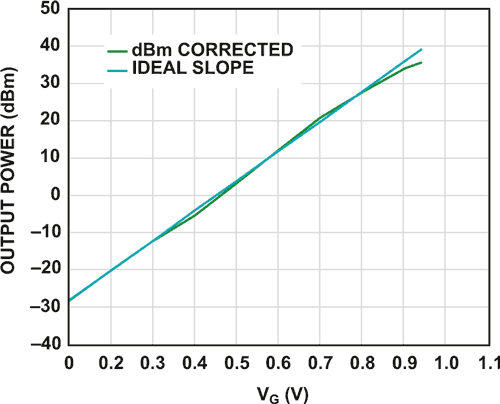Figure 13. Padded, unfiltered, output power results. Without the filter, system exhibits a P1dB point at 34 dBm. FTEST = 14.0956 MHz.

The gain range can be improved with better filtering at the DDS output, as well as by reducing system noise. Figure 14 shows the same measurements with a filter. The filtered output does not suffer the same P1dB level, producing a full +36 dBm output into a 50-Ω load. The overall gain linearity is better (≤0.65 dB), with errors only present around midscale.

If even higher output powers were required for particular modes of operation, multiple output amplifiers could be used to drive a specialty transformer for the given application.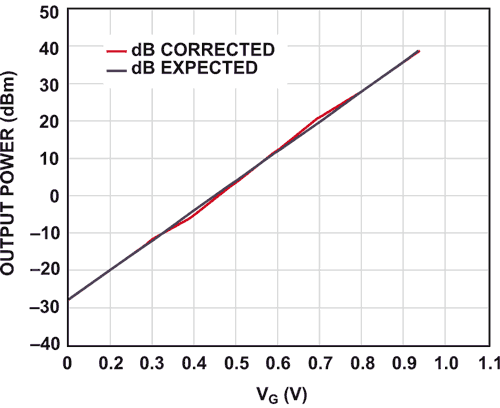Figure 14. Measured output with a 5th order low-pass filter (fC = 20 MHz). FTEST = 14.0956 MHz.

Alternatively, the design methods described here could be applied to lower supply systems, as long as the methods described here are used within the constraints of the alternate designs.

Note that the upper end of the measurement stopped at VG = 0.9375 V as a result of accumulated input attenuation and gain errors. This can be resolved by trimming the initial attenuation network to account for the total system errors. Once corrected, the total system gain range increased to 74 dB.

Conclusion

By pairing a high-performance VGA and a high-performance, high-output CFA, a simple front end for a new generation of signal generator can be constructed. Total PC board area and cost are reduced due to the high integration of these parts.

For additional versatility, a log amplifier, such as the AD8310 , can be used in a closed-loop feedback system. With this addition, working in conjunction with a DDS like the AD9834C, the designer can incorporate various forms of envelope modulation, such as frequency shift keying (FSK), on-off keying (OOK), and phase-shift keying (PSK), as an intrinsic feature, offering countless options through the novel use of two fundamental blocks.

References

1. Current Feedback Amplifiers.
2. Log Amps/Detectors.
3. Signal Synthesis.
4. Variable Gain Amplifiers (VGA).
5. MT-034 Tutorial. Current Feedback (CFB) Op Amps.
6. MT-057 Tutorial. High Speed Current Feedback Op Amps.
7. MT-060 Tutorial. Choosing Between Voltage Feedback and Current Feedback Op Amps.
8. MT-072 Tutorial. Precision Variable Gain Amplifiers.
9. MT-073 Tutorial. High Speed Variable Gain Amplifiers.

analog.com